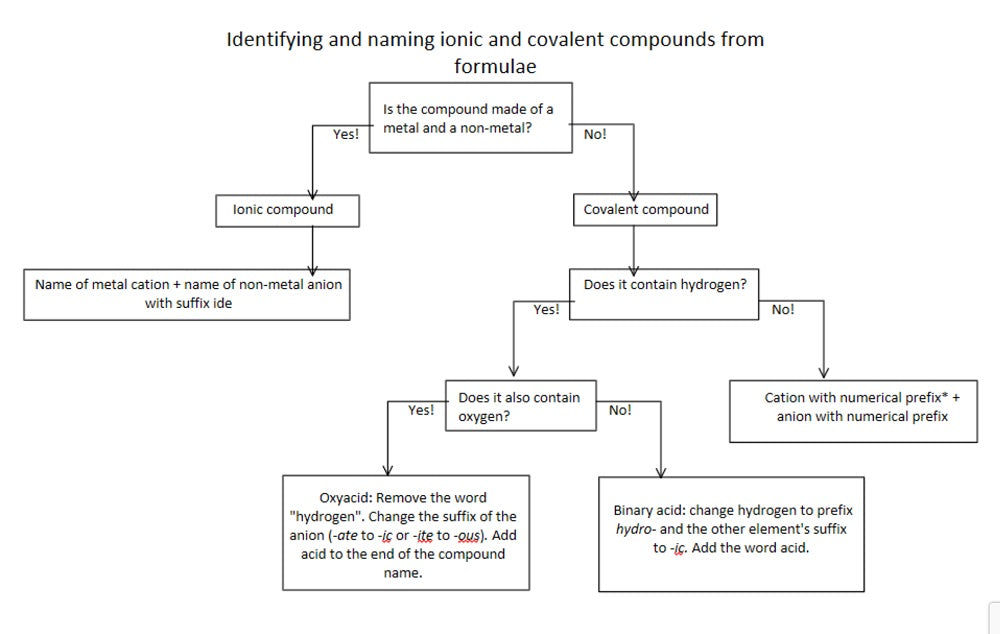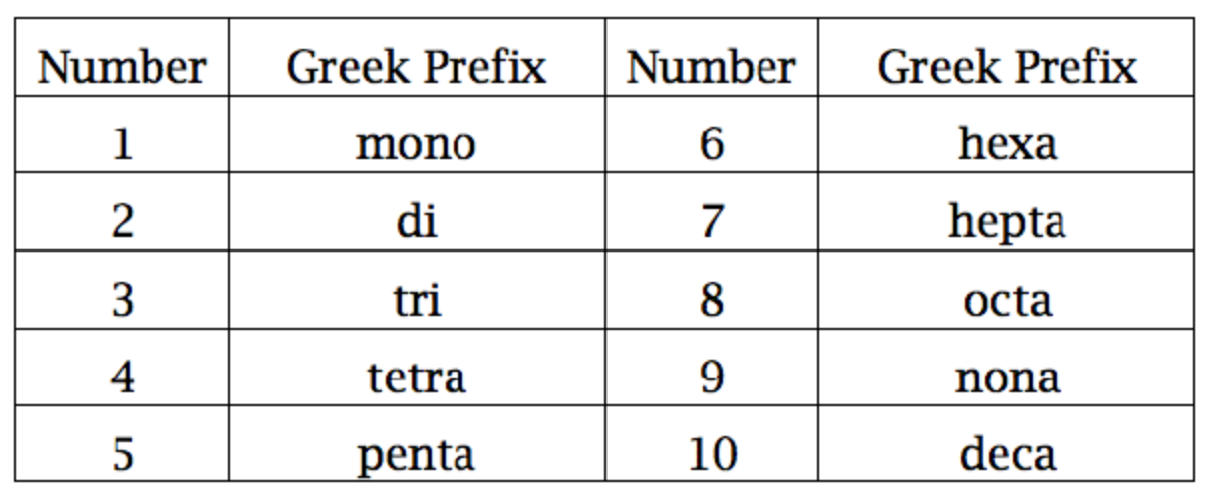# How to Name Ionic and Covalent Compounds

Want to know how to name ionic compounds and covalent compounds? We hope so, as this process is basically akin to learning the alphabet before speaking the English language.

## How to Get the Name of a Compound from the Formula

Naming chemical compounds from the molecular or ionic formula can be intimidating, but if you grab a Periodic Table and follow a few simple rules for naming compounds, you'll be a master at naming compounds in no time at all. Here's how...

Start by examining the chart below and visualizing how we convert "compound formulas" into "compound names."## Naming Ionic Compounds When Given the Formula

The first thing we need to do is figure out what kind of compound we are working with, as ionic and covalent compounds have very different naming systems.

Ionic compounds are made up of a metal cation (named first) and a non-metal anion (named second). If the first element you "see" in the compound is found on the left side of the periodic table (i.e. left of the metalloid staircase), it is a metal and the compound is "ionic."

When naming ionic compounds, we never indicate the ratio of component elements in the name - that is for covalent compounds only.

Examples

NaCl = Sodium Chloride

KBr = Potassium Bromide

Mg3N2 = Magnesium Nitride

If only it were that easy.  "What do you mean?" you ask… Well it's also possible to have ionic compounds that contain polyatomic ions.

These are usually negatively charged "clumps" or "chunks" of ions that form bonds to the oppositely charged ion (usually a cation).

Follow the same naming conventions as with monatomic ions, except for the step wherein the suffix is changed to -ide. Instead, retain the suffix of the polyatomic ion. Three examples are below.

Examples:

CaSO4 = Calcium Sulfate

Mg3(PO4)2 = Magnesium Phosphate

Al(OH)3 = Aluminum HydroxideSome metal cations can have multiple charges. These are usually the transition metals. For example, copper can be Cu
+1 or Cu+2. Naming compounds that contain metals with multiple charges is done much the same way as naming other ionic compounds with one crucial difference: the charge of the metal is included in the compound name using roman numerals. See the examples here...

Examples:

FeO= iron (II) oxide

Cu3N = copper (I) nitride

Hg2O = mercury (I) oxide

In earlier years, chemists named ionic compounds containing metals with multiple charges using the suffixes -ic and -ous. This is not current practice in most areas of the scientific community and we will not discuss it here.

## Naming Covalent Compounds (or "Molecular Compounds") When Given the Formula

Covalent compounds, often called "molecular compounds," consist of two non-metals; a non-metal / non-metal pair. These elements will be found on the far right side of the periodic table - to the right of the metalloid staircase.

The simplest form of a covalent compound would be a non-metal / non-metal pair made of two single elements (NO for example). In this instance the two elements are bonded in a 1:1 ratio.

In a covalent compound of this type, we'd use the name of the first element, then mono [name of second element] ide. Only use numerical prefixes for the first element when the molecule contains more than one of that atom.Examples:

SF6 = Sulfur Hexafluoride

CO2 = Carbon Dioxide

N2O4 = Dinitrogen Tetroxide

## How to Name Binary Acids and Oxyacids in General Chemistry

Acids are a type of covalent compound that release hydrogen ions when dissolved in water. Binary acids are composed of hydrogen and one other element, while oxyacids contain hydrogen, oxygen, and another element.

Translating binary acids from formula to name will end up in the format hydro[root of anion]ic acid.

Translating oxyacids from formula to name involves dropping the word hydrogen completely, replacing the anion’s suffix, and adding acid. The oxyacid suffix replacements are -ate becomes -ic and -ite becomes -ous.

Note that the definition of acid includes dissolved in water. That means all acids, binary and oxyacid, will be aqueous, or dissolved in water. This will be marked by the subscript "aq," as shown in the examples below.Examples:

HF (aq) = Hydrofluoric Acid

HCl (aq) = Hydrochloric Acid

H2SO4 (aq) = Sulfuric Acid

H2SO3 (aq) = Sulfurous Acid

H2CO3 (aq) = Carbonic Acid

## Finding Chemical Formulas from Names of Chemical Compounds

We have discovered how to change chemical formulae into names of chemicals. What about converting compound names into compound formulas?

The process is not much different.

For ionic compounds, start by listing the component ions. Write the charges on the ions. Find the ratio of positive ions to negative ions that results in a net charge of zero. This is called the "criss-cross" or "crossover" method.

For covalent compounds the process is simpler, as the ratio of elements is indicated by the numerical prefixes.

Examples:

Sodium Fluoride = NaF

Copper (II) Nitrate = Cu(NO₃)₂

Carbon Dioxide = CO₂